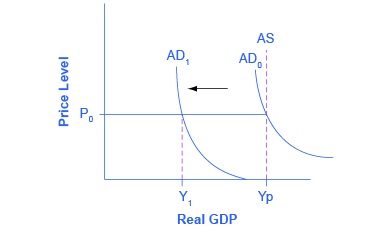## Real Aggregate Supply in the Income-Expenditure Model

### Learning Objectives

• Explain aggregate supply in the income-expenditure model and how the income-expenditure model correlates to the AD-AS model

We observed earlier the income-expenditure model doesn’t explicitly discuss aggregate supply, but it’s straightforward to add that, if we think of the aggregate supply curve as answering the question: how do producers respond to a change in aggregate demand? Recall Figure 1 below from our earlier discussion of aggregate demand in the Keynesian model. Figure 1 shows the pure Keynesian AD-AS model. Let’s think about how this corresponds to the income-expenditure model.Figure 1. The Pure Keynesian AD–AS Model. The Keynesian View of the AD–AS Model uses an AS curve which is horizontal at levels of output below potential and vertical at potential output. Thus, changes in AD only affect GDP when below potential output, but only affect the price level when at potential output.

The pure Keynesian AD-AS model assumes that for any level of GDP below potential, any change in AD affects real GDP, but not the price level. This corresponds to the Keynesian Cross diagram to the left of the Potential GDP line. But to the right of the Potential GDP line, any change in AD affects the price level but not real GDP. This makes sense since potential GDP means all resources are fully employed so it’s not possible to produce more output. Thus, any increase in AD can only lead to inflation. Let’s redraw the Keynesian Cross diagram to illustrate this (Figure 2). Ep plays the role of aggregate demand, and the income equals expenditure line plays the role of aggregate supply.  But once we reach potential GDP, AS becomes vertical, just as it does in the traditional AD-AS model shown in Figure 1.

Figure 2 (Interactive Graph). The Real Aggregate Supply (RAS) Curve.

This version of the Keynesian Cross works exactly like the original version for changes in aggregate expenditure. But it also allows for positive and negative supply shocks which show up as shifts in real aggregate supply due to changes in resource prices, productivity, etc.

## Supply Shocks

Suppose there is a positive supply shock, for example, an increase in the labor supply. A larger labor supply means the economy can produce more output, so the level of potential GDP (Yp) shifts to the right. As a result, an increase in Ep doesn’t cause higher prices since the increased Yp provides room for the economy to grow.  Thus, increased Ep leads to increased Y.  Of course, if Ep increases enough, the economy would pass Yp and the increased spending would cause inflation, but no additional real GDP.

## Contribute!

Did you have an idea for improving this content? We’d love your input.